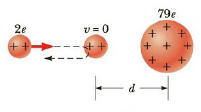Chapter 16, Problem 23P

Chapter
Section
Textbook Problem

In Rutherford’s famous scattering experiments that led to the planetary model of the atom, alpha panicles (haring charges of +2e and masses of 6.64 × 10−27 kg) were fired toward a gold nucleus with charge +79e. An alpha particle, initially very far from the gold nucleus, is fired at 2.00 × 107 m/s directly toward the nucleus, as in Figure P16.23. How close does the alpha particle get to the gold nucleus before turning around? Assume the gold nucleus remains stationary.Figure P16.23

To determine
The distance of closest approach.

Explanation

Given info: The initial speed of alpha particle is 2.00×107m/s

Explanation:

From the law of conservation of momentum,

12mαvα2=ke(79e)(2e)r

• mα is the mass of alpha particle.
• vα is the speed of alpha particle.
• e is the elementary charge.
• r is the distance of closest approach.

On Re-arranging,

r=316kee2mαvα2

Substitute 1.6×1019C for e, 8.99×109 Nm2/C2 for ke , 2

Still sussing out bartleby?

Check out a sample textbook solution.

See a sample solution

The Solution to Your Study Problems

Bartleby provides explanations to thousands of textbook problems written by our experts, many with advanced degrees!

Get Started

Find more solutions based on key concepts